Latest Banking jobs   »

# Reasoning Ability Quiz For IBPS Clerk Prelims 2022- 1st August

Directions (1-5): Study the following series carefully and answer the questions accordingly.

MEAN   WHEN   ROSE   MOCK   TIRE

Q1. When the first and the third letters of each word are interchanged, then how many meaningful word(s) will be formed?
(a) Four
(b) One
(c) Three
(d) Two
(e) None

Q2. If each consonant is changed to the next letter according to alphabetical series, then how many words will contain more than two vowels?
(a) Four
(b) One
(c) Three
(d) Two
(e) None

Q3. If the words are arranged according to the alphabetical series from left to right, then which of the following word will be second from the left end?
(a) MEAN
(b) WHEN
(c) TIRE
(d) ROSE
(e) MOCK

Q4. If the letters in each word are arranged according to the alphabetical series from left to right within the words, then how many words will remain the same?
(a) Four
(b) One
(c) Three
(d) Two
(e) None

Q5. If the third and the fourth letters of each word are interchanged, then how many words will end with a consonant?
(a) Four
(b) Two
(c) Three
(d) One
(e) None of these

Directions (6-10): Study the following series carefully and answer the questions accordingly.

C * 7 % D 5 A 2 # G 9 > K 3 6 P @ 8 U M < B 4 S \$ E

Q6. How many such letters are immediately preceded by a number and immediately followed by a symbol?
(a) More than three
(b) Two
(c) Three
(d) One
(e) None

Q7. How many such symbols are immediately followed by a number and immediately preceded by a consonant?
(a) Two
(b) One
(c) Four
(d) Three
(e) None

Q8. Which of the following is the 6th element to the right of 13th element from the left?
(a) M
(b) 8
(C) @
(d) U
(e) None of these

Q9. If all the numbers are dropped from the given series, then which of the following element will be 16th from the right end?
(a) A
(b) D
(c) *
(d) %
(e) None of these

Q10. Which of the following element is 5th to the right of 17th element from the right end?
(a) P
(b) 3
(c) 6
(d) 4
(e) None of these

Directions (11-12): Study the following information carefully and answer the questions accordingly.
Point B is 10m south of point L. Point C is 15m east of point B. Point C is 18 m north of point J. Point E is 20m west of point J. Point F is 20m north of point E.

Q11. If point Y is 8m north to the Point F, then point L is how far and in which direction with respect to point Y?
(a) 5m, south
(b) 5m, east
(c) 5m, west
(d) 5m, north
(e) None of these

Q12. Point F is in which direction with respect to C?
(a) South-west
(b) South-east
(c) North-west
(d) North-east
(e) None of these

Directions (13-15): Study the following information carefully and answer the questions accordingly.
Neeraj started his journey from his house to his office. He walks 15m towards north direction and stops at point A. From there, he takes two consecutive right turns of 10m each and stops at point B. From there he takes two consecutive left turns of 8m each and stops at point C. From there, he walks 18m to his right and stops at point D. Neeraj’s office is in south of point D and east of point B.

Q13. What is the total distance from Point B to Point D?
(a) 35m
(b) 34m
(c) 37m
(d) 38m
(e) None of the above

Q14. What is the direction of D with respect to A?
(a) North east
(b) South east
(c) North west
(d) South west
(e) None of the above

Q15. What is the distance between point D and his Office?
(a) 10m
(b) 11m
(c) 12m
(d) 8m
(e) None of the above

Solutions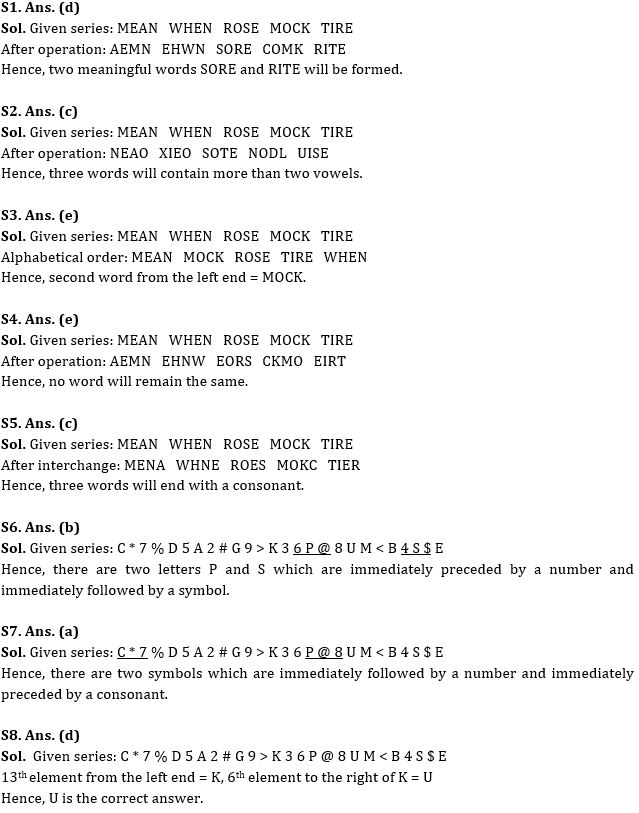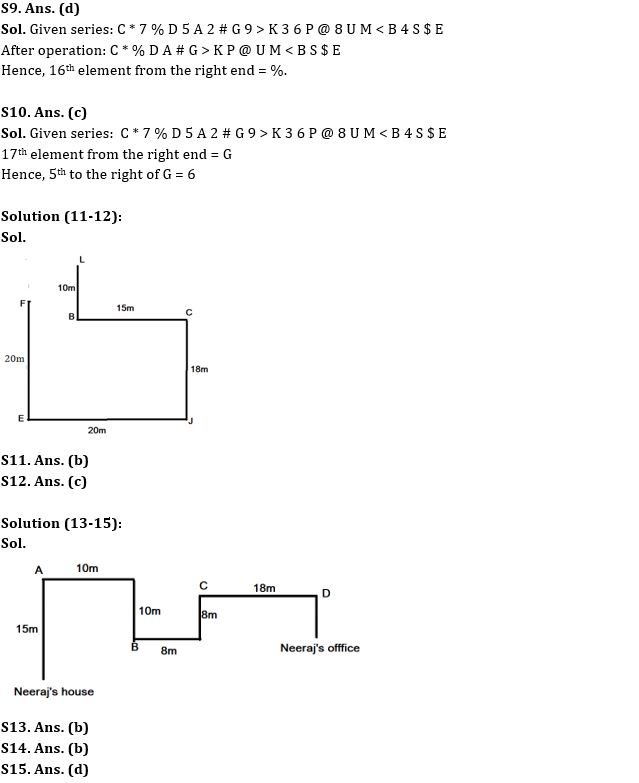#### Congratulations!Incorrect details? Fill the form again here

•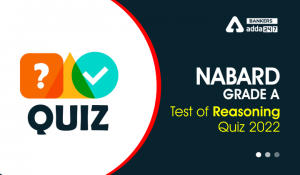Reasoning Ability Quiz For NABARD Grade ...
•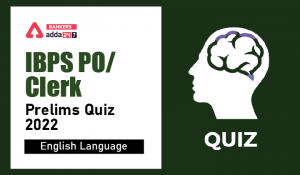English Quizzes For IBPS Clerk/PO Prelim...
•English Quizzes For IBPS Clerk/PO Prelim...
•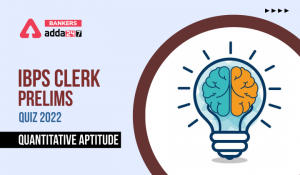Quantitative Aptitude Quiz For IBPS Cler...
•Quantitative Aptitude Quiz For IBPS Cler...
•Reasoning Ability Quiz For IBPS Clerk/PO...